# How to Calculate Number of Days in Microsoft Excel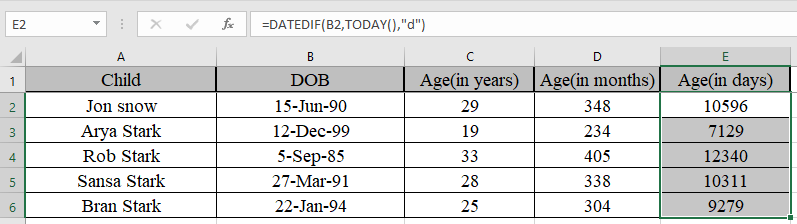In this article, we will learn How to Calculate Number of Days in Microsoft Excel.

How can you calculate days between two dates ?

There could be a simple way to just subtract the latest date from the older date. Or you can use the Excel DATEDIF function. This function sometimes doesn't show up. You have to type it as a whole text. You can choose the today's date using the today function explained in the example to calculate the age of a person in days, months or years in Excel.

DATEDIF Function in Excel

DATEDIF function is an excel function to get the complete calendar period between the two dates. DATEDIF function in excel  to get the age of a person. Basically it's a subtraction function for dates in excel as this function returns the number expressed as calendar period.

DATEDIF function returns the number of years, months and days between the given two dates in Excel.

Syntax:

 =DATEDIF(start_date, end_date, unit)

Start_date : First date in a valid excel format

end_date : Second date in a valid excel format

Unit : unit in quotes

“y” gets the Years between dates

“m” gets the between dates

“d” gets the days between dates

"md"    gets the days between dates, ignoring months and years

"ym"    gets the months between dates, ignoring days and years

"yd"    gets the days between dates, ignoring years

 Unit Purpose Meaning d days Complete calendar days between the days m months Complete calendar months between the days y years Complete calendar years between the days md Days without months and years Complete calendar days between the days as if the dates were of the same month & year ym Months without years Complete calendar months between the days as if the dates were of the same year yd Days without years Complete calendar days between the days as if the dates were of the same year

Unit argument is very important.

Example :

All of these might be confusing to understand. Let's understand how to use the function using an example.

Here we have some examples to test the formula. Here given the start date and end date, we need to calculate the difference in days between dates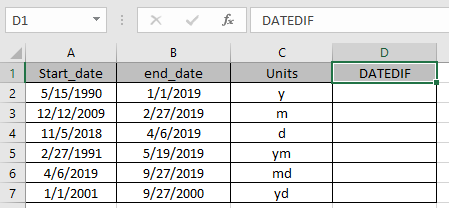Use the Formula:

 =DATEDIF (A2, B2, C2)

A2 : first date argument given as cell reference

B2 : Second date argument given as cell reference

C2 : specified unit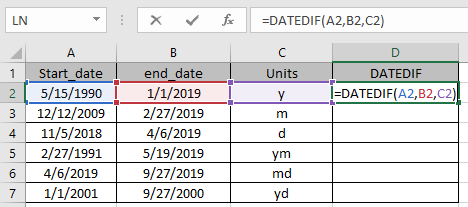Marked boxes while using the function shows us that argument to the function given as cell reference.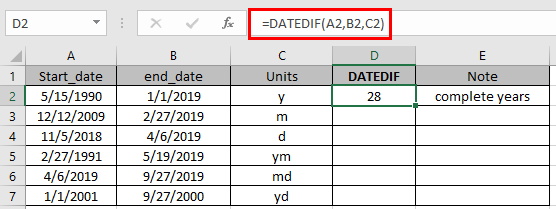The function returns the complete calendar years between the dates using the “y”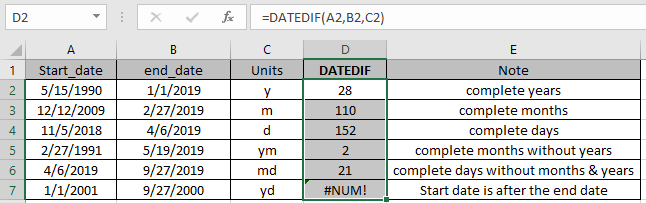As you can see the function returns the values.

Note:

1. The date in excel must be in valid format
2. Unit argument to the function can be given as cell reference or directly to the function in double quotes.
3. Start_date must be before the end_date or else the function will give #NUM! error
4. The function gives #VALUE! Error if the date is not valid date.

One of the most used example of the function is to get the age of a person in excel.

Here below is an example to get the age of a person in excel using the DATEDIF function.

DATEDIF function is combined with TODAY function for the purpose.

TODAY function in Excel is used to generate today’s date in a cell.

Syntax:

 =Today()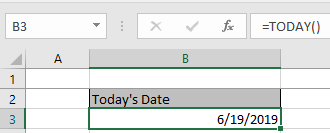Let’s get this with an example shown below.

Here we need to calculate the age of Ned Stark’s children.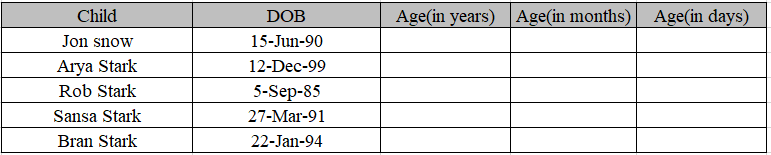Use the formula to get the age of children.

Formula:

 =DATEDIF(C4,TODAY(),"y")

Date of birth is the start date in the C4 cell.

Today() function is used to get Today’s date as an end date.

“Y” to get the age in years.

 Note: Here we are using Today as an end date. You can use end date as your choice.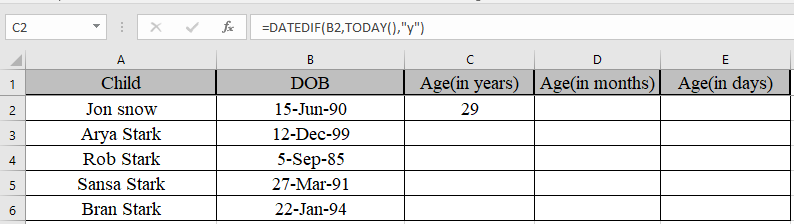We got the age of Jon Snow using DATEDIF function

Copy the formula in other cells, select the cells taking the first cell where the formula is already applied, use shortcut key Ctrl + D to find the age of rest of the children.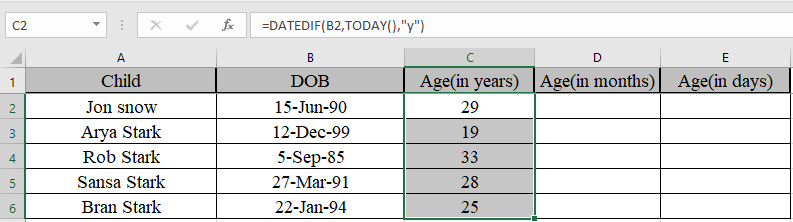Similarly, we will find out months and days using the same function but different unit.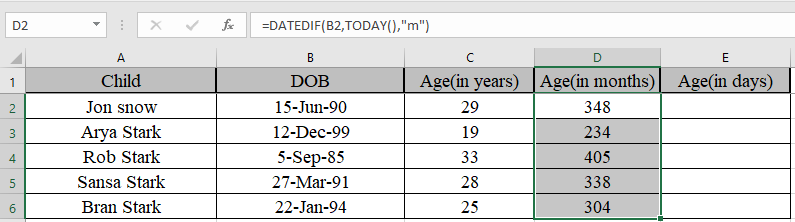We got the age in monthsWe got the age in days

Now Some of you are wondering if we need to find the years, months and days between the two dates.

Use the formula to get the years, months and days together

 =DATEDIF(B2,TODAY(),"y") & "y " & DATEDIF(B2,TODAY(),"ym")&"m " & DATEDIF(B2,TODAY(),"md") & "d"

Explanation:

DATEDIF(B2,TODAY(),"y") & "y " denotes years between dates with string “y ” attached to the number

DATEDIF(B2,TODAY(),"ym")&"m " denotes months between dates, ignoring days and years with string “m ” attached to the number.

DATEDIF(B2,TODAY(),"md") & "d" denotes days between dates, ignoring months and years with string “d” attached to the number.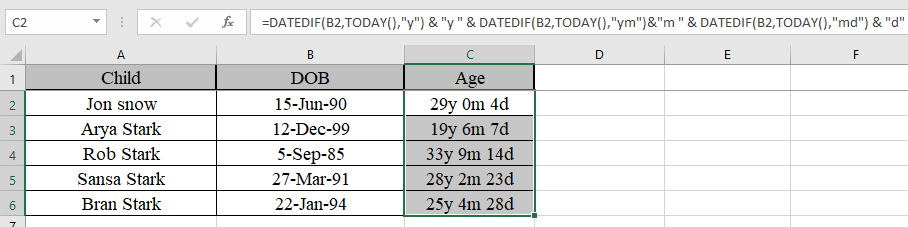As you can see their age now. You can use this function to calculate the number of days in service in Excel.

Hope this article about How to Calculate Number of Days in Microsoft Excel is explanatory. Find more articles on calculating date and time values and related Excel formulas here. If you liked our blogs, share it with your friends on Facebook. And also you can follow us on Twitter and Facebook. We would love to hear from you, do let us know how we can improve, complement or innovate our work and make it better for you. Write to us at info@exceltip.com.

Related Articles :

How to use the WORKDAY function in Excel : Returns a workday date value after days added to the start_date using the WORKDAYS function in Excel.

How to use the NETWORKDAYS function in Excel : Returns the working days between the two given dates using the NETWORKDAYS function.

Count holidays between dates in Excel : count non working days between the two given dates using the DATEDIF and NETWORKDAYS function in Excel.

Extract days between dates ignoring years in Excel : count the days between the two dates using different functions and mathematical operation in Excel

Count Birth Dates By Month in Excel : count the number of dates lying in a particular month using the SUMPRODUCT and MONTH function in Excel.

SUM price by weekdays in Excel : sum price values corresponding to the number of dates in a particular weekday using the SUMPRODUCT and WEEKDAY function in Excel.

Popular Articles :

How to use the IF Function in Excel : The IF statement in Excel checks the condition and returns a specific value if the condition is TRUE or returns another specific value if FALSE.

How to use the VLOOKUP Function in Excel : This is one of the most used and popular functions of excel that is used to lookup value from different ranges and sheets.

How to Use SUMIF Function in Excel : This is another dashboard essential function. This helps you sum up values on specific conditions.

How to use the COUNTIF Function in Excel : Count values with conditions using this amazing function. You don't need to filter your data to count specific values. Countif function is essential to prepare your dashboard.

Terms and Conditions of use

The applications/code on this site are distributed as is and without warranties or liability. In no event shall the owner of the copyrights, or the authors of the applications/code be liable for any loss of profit, any problems or any damage resulting from the use or evaluation of the applications/code.